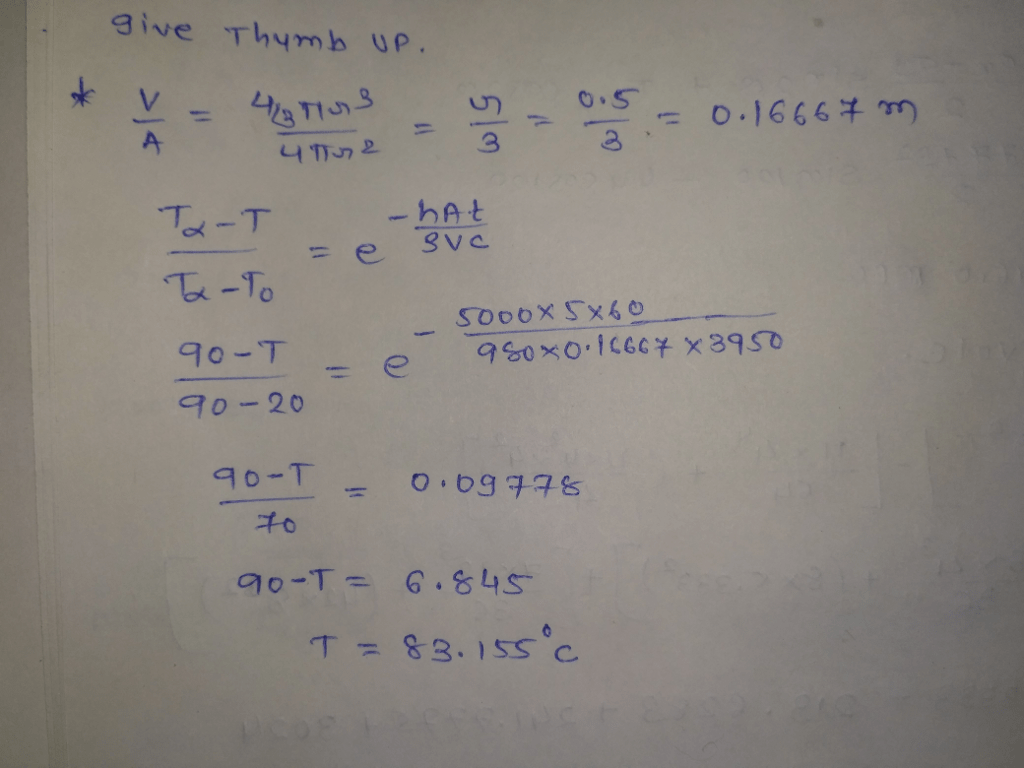# Calculate the temperature of tomato juice (density=980kg/m3) in a steam jacketed kettle after 5 minutes of heating. The radius of the kettle is 0.5m, the convective heat transfer coefficient in the steam jacket is 5000W/m2C. The inside surface temperature of the kettle is 90C. The initial temperature of tomato juice is 20C. Assume specific heat of the tomato juice is 3.95kJ/kgC. The thermal conducriviry of the kettle is known to be k=10000W/mC

Question-AnswerCategory: Heat And Mass TransferCalculate the temperature of tomato juice (density=980kg/m3) in a steam jacketed kettle after 5 minutes of heating. The radius of the kettle is 0.5m, the convective heat transfer coefficient in the steam jacket is 5000W/m2C. The inside surface temperature of the kettle is 90C. The initial temperature of tomato juice is 20C. Assume specific heat of the tomato juice is 3.95kJ/kgC. The thermal conducriviry of the kettle is known to be k=10000W/mC

Calculate the temperature of tomato juice (density=980kg/m3) in a steam jacketed kettle after 5 minutes of heating. The radius of the kettle is 0.5m, the convective heat transfer coefficient in the steam jacket is 5000W/m2C. The inside surface temperature of the kettle is 90C. The initial temperature of tomato juice is 20C. Assume specific heat of the tomato juice is 3.95kJ/kgC. The thermal conducriviry of the kettle is known to be k=10000W/mC